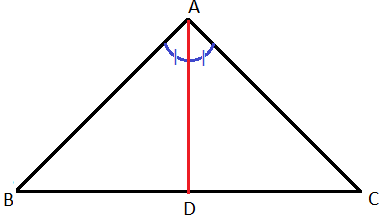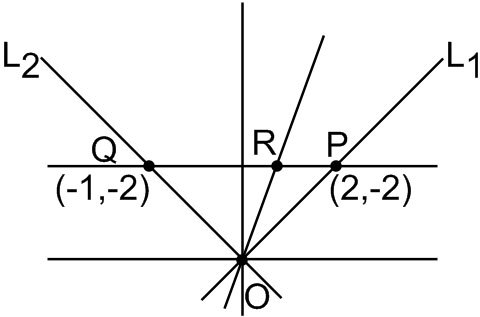#### The linesintersect the lineatrespectively. The bisector of the acute angle betweenStatement-1 : The ratioStatement-2 : In any triangle, bisector of an angle divides the triangle into two similar triangles. Option 1) Statement-1 is true, Statement-2 is false. Option 2) Statement-1 is false, Statement-2 is true. Option 3) Statement-1 is true, Statement-2 is true; Statement-2 is a correct explanation for Statement-1. Option 4) Statement-1 is true, Statement-2 is true; Statement-2 is not a correct explanation for Statement-1.As we learnt in

Angle Bisector -

A line segment that bisects an angle of a triangle.

- whereinFor point P: y-x=0 and y+2=0

So P is (2,-2)

For point Q: 2x+y=0 and y+2=0

So Q is (-1, -2)But statement 2 is false

Option 1)

Statement-1 is true, Statement-2 is false.

This option is correct

Option 2)

Statement-1 is false, Statement-2 is true.

This option is incorrect

Option 3)

Statement-1 is true, Statement-2 is true; Statement-2 is a correct explanation for Statement-1.

This option is incorrect

Option 4)

Statement-1 is true, Statement-2 is true; Statement-2 is not a correct explanation for Statement-1.

This option is incorrect

#### divya.saini